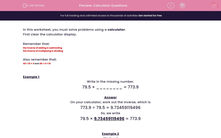# Calculator Questions

In this worksheet, students solve number problems using a calculator.This content is premium and exclusive to EdPlace subscribers.Key stage:  KS 2

Curriculum topic:   Maths and Numerical Reasoning

Curriculum subtopic:   Mixed Problems

Difficulty level:#### Worksheet Overview

In this worksheet, you must solve problems using a calculator.

First clear the calculator display.

Remember that:

• the inverse of adding is subtracting
• the inverse of multiplying is dividing

Also remember that:

• 40 ÷ 10 = 4 and 40 ÷ 4 = 10

Example 1

Write in the missing number.

79.5 × ________ = 773.9

On your calculator, work out the inverse, which is

773.9 ÷ 79.5 = 9.73459119496

So, we write

79.5 × 9.73459119496 = 773.9

Example 2

Work out

45% of 3404

45% is 45/100 which is 0.45 as a decimal.

So, on the calculator, we work out

0.45 × 3404 = 1531.8

### What is EdPlace?

We're your National Curriculum aligned online education content provider helping each child succeed in English, maths and science from year 1 to GCSE. With an EdPlace account you’ll be able to track and measure progress, helping each child achieve their best. We build confidence and attainment by personalising each child’s learning at a level that suits them.

Get started